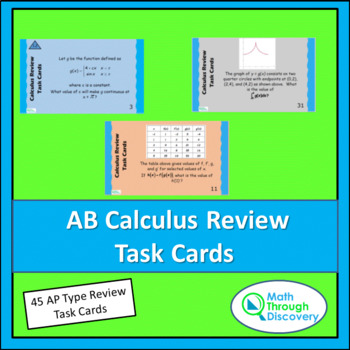# AB Calculus Review Task CardsSubject
Resource Type
Format
PDF (1 MB|58 pages)
\$9.00
\$9.00

#### Also included in

1. These two sets of cards (AB and BC Calculus Review Task Cards) each contain 45 questions that review the entire two courses. The AB cards are separated into three categories based on the three big ideas: Limits, Derivatives, and Antiderivatives.The BC cards are separated into four categories based o
\$16.20
\$18.00
Save \$1.80

### Description

This set of 45 task cards can be used to review for an end of the year calculus exam or for the Calculus AB exam.

In the new curriculum framework for Calculus AB divides the topics by their Big Idea and their Learning Objective. So these 45 cards have also been divided by those same two categories.

You can separate the cards into the three groups by their Big Idea:

• Blue cards are on the Big Idea of Limits.

• Salmon colored cards are on the Big Idea of Derivatives.

• Gray colored cards are on the Big Idea of Integration and the Fundamental Theorem of Calculus.

In addition each card has a blue triangle with the learning objective identified with a number:

• 1.1 Determining limits of function

• 1.1 Estimating limits of a function

• 1.2 Analyzing functions for intervals of continuity or points of discontinuity

• 1.2 Determining the applicability of important theorems using continuity

• 2.1 Calculating derivatives

• 2.1 Determining high order derivatives

• 2.1 Identifying the derivative of a function as the limit of a difference quotient

• 2.1 Calculating derivatives and higher order derivatives

• 2.2 Recognizing the connection between differentiability and continuity

• 2.2 Using derivatives to analyze properties of a function

• 2.3 Solving problems involving the slope of a tangent line

• 2.3 Solving problems involving related rates, optimization, and rectilinear motion

• 2.3 Solving problems involving reates of change in applied contexts

• 2.3 Interpreting the meaning of a derivative within a problem

• 2.3 Verify solutions to differential equations

• 2.3 Estimating solutions to differential equations

• 2.4 Applying the Mean Value Theorem to describe the behavior of a function over an interval

• 3.2 Approximating a definite integral

• 3.2 Calculating a definite integral using areas and properties of definite integrals

• 3.2 Calculating a definite integral using areas and properties of definite integrals

• 3.2 Interpreting the definite integral as the limit of a Riemann sum

• 3.3 Evaluating definite integrals

• 3.3 Analyzing functions defined by integrals

• 3.4 Applying definite integrals to problems involving area and volume

• 3.4 Applying definite integrals to problems involving the average value of a function

• 3.4 Using the definite integral to solve problems in various contexts

• 3.4 Applying definite integrals to problems involving motion

• 3.4 Interpreting the meaning of a definite integral within a problem

• 3.5 Analyzing differential equations to obtain general and specific solutions

A complete set of solutions is included with the cards. On the solution key is the card number, the questions, the learning objective, and the solution.

The questions have been modeled after questions used on AP practice exams.

When it is appropriate to use the graphing calculator to answer a question, the card clearly states that it is a GRAPHING CALCULATOR QUESTION.

When similar questions are given on the AP exam, students have on an average two minutes to complete a non-graphing calculator question and three minutes to complete a calculator active question.

This is phenomenal! I am so glad there are more Calculus resources being posted!

Used these as a final review before the AP test. Nice little refresher.

A great resource for review.

Loved using these for review for the AP Exam!

I plan to use these cards to start the AP Review next week! Thanks!

This identical product is also available in digital format as three google forms:

Total Pages
58 pages
Included
Teaching Duration
45 minutes
Report this Resource to TpT
Reported resources will be reviewed by our team. Report this resource to let us know if this resource violates TpT’s content guidelines.